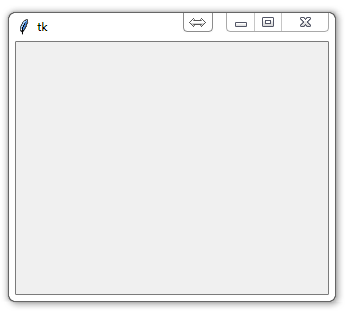## Tkinter intro

In this video, we begin discussion of the tkinter module. The tkinter module is a wrapper around tk, which is a wrapper around tcl, which is what is used to create windows and graphical user interfaces. Here, we show how simple it is to create a very basic window in just 8 lines. We get a window that we can resize, minimize, maximize, and close! The tkinter module's purpose is to generate GUIs. Python is not very popularly used for this purpose, but it is more than capable of doing it.

Let's walk through each step to making a tkinter window:

Simple enough, just import everything from tkinter.

```from tkinter import *
```

Here, we are creating our class, Window, and inheriting from the Frame class. Frame is a class from the tkinter module. (see Lib/tkinter/__init__)

Then we define the settings upon initialization. This is the master widget.

```class Window(Frame):

def __init__(self, master=None):
Frame.__init__(self, master)
self.master = master
```

The above is really all we need to do to get a window instance started.

Root window created. Here, that would be the only window, but you can later have windows within windows.

```root = Tk()
```

Then we actually create the instance.

```app = Window(root)
```

Finally, show it and begin the mainloop.

```root.mainloop()
```

The above code put together should spawn you a window that looks like:Pretty neat, huh? Obviously there is much more to cover.

The next tutorial:• Python Introduction

• Print Function and Strings

• Math with Python

• Variables Python Tutorial

• While Loop Python Tutorial

• For Loop Python Tutorial

• If Statement Python Tutorial

• If Else Python Tutorial

• If Elif Else Python Tutorial

• Functions Python Tutorial

• Function Parameters Python Tutorial

• Function Parameter Defaults Python Tutorial

• Global and Local Variables Python Tutorial

• Installing Modules Python Tutorial

• How to download and install Python Packages and Modules with Pip

• Common Errors Python Tutorial

• Writing to a File Python Tutorial

• Appending Files Python Tutorial

• Reading from Files Python Tutorial

• Classes Python Tutorial

• Frequently asked Questions Python Tutorial

• Getting User Input Python Tutorial

• Statistics Module Python Tutorial

• Module import Syntax Python Tutorial

• Making your own Modules Python Tutorial

• Python Lists vs Tuples

• List Manipulation Python Tutorial

• Multi-dimensional lists Python Tutorial

• Reading CSV files in Python

• Try and Except Error handling Python Tutorial

• Multi-Line printing Python Tutorial

• Python dictionaries

• Built in functions Python Tutorial

• OS Module Python Tutorial

• SYS module Python Tutorial

• Python urllib tutorial for Accessing the Internet

• Regular Expressions with re Python Tutorial

• How to Parse a Website with regex and urllib Python Tutorial

• Tkinter intro
• Tkinter buttons

• Tkinter event handling

• Tkinter images, text, and conclusion

• CX_Freeze Python Tutorial

• The Subprocess Module Python Tutorial

• Matplotlib Crash Course Python Tutorial

• Python ftplib Tutorial

• Sockets with Python Intro

• Simple Port Scanner with Sockets